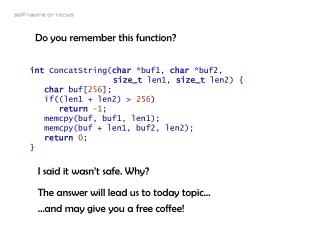DownloadDownload Presentationint ConcatString( char *buf1, char *buf2,

# int ConcatString( char *buf1, char *buf2,

Download Presentation## int ConcatString( char *buf1, char *buf2,

- - - - - - - - - - - - - - - - - - - - - - - - - - - E N D - - - - - - - - - - - - - - - - - - - - - - - - - - -
##### Presentation Transcript

1. Do you remember this function? int ConcatString(char *buf1, char *buf2, size_t len1, size_t len2) { char buf; if((len1 + len2) > 256) return-1; memcpy(buf, buf1, len1); memcpy(buf + len1, buf2, len2); return0; } I said it wasn’t safe. Why? The answer will lead us to today topic… …and may give you a free coffee!

2. Software attacks Integer overflow

3. (size_t)0xFFFFFFFF + (size_t)0x2 = 0x1 or, if you prefer, (size_t)4294967295 + (size_t)2 = 1 The problem The previous function is not safe, because integer types have a finite precision. So the previous function could have an integer overflow, which in turn (in this example) can lead to a buffer overflow

4. Integer overflow Integer overflows occur when a larger integer is needed to accurately represent the results of an arithmetic operation. Let's look at a simple example using an unsigned char (8-bit) integer type: unsigned char a = 255;unsigned char b = 2;unsigned char result = a + b; When compiled and run, we get the following binary representation: 11111111 (a)+00000010 (b) --------100000001 (result)

5. Integer overflow Ashcraft & Engler [IEEE S&P 2002]: “Many programmers appear to view integers as having arbitrary precision, rather than being fixed-sized quantities operated on with modulo arithmetic.” bool DoSomething(const char* server){unsigned char namelen = strlen(server) + 2; if(namelen < 255){char* UncName = malloc(namelen); if(UncName != 0) sprintf(UncName, "\\\\%s", server); //do more things here}}

6. The solution Solving integer overflow problems is not easy:they are very subtle int ConcatString(char *buf1, char *buf2, size_t len1, size_t len2) { char buf; if((len1 < 256 - len2) && (len2 < 256 – len1)) return-1; memcpy(buf, buf1, len1); memcpy(buf + len1, buf2, len2); return0; }

7. The solution For multiplication, in mathematical terms, the general problem is:A * B > MAX_INT => Error! In order to keep your test from depending on an overflow, we can rewrite it as follows:A > MAX_INT/B => Error! For addition, the unsigned case is relatively easy:A + B > MAX_INT Error! In order to keep your test from depending on an overflow, we can rewrite it as follows:A > MAX_INT – B Error!

8. The solution Testing a signed addition operation is considerably more difficult. We actually have four cases—both numbers are positive, both numbers are negative, and two mixed-sign cases: if(!((rhs ^ lhs) < 0)) //test for +/- combo{//either two negatives, or 2 positivesif(rhs < 0){//two negativesif(lhs < MinInt() - rhs) //remember rhs < 0throw ERROR_ARITHMETIC_OVERFLOW;}else{//two positivesif(MaxInt() - lhs < rhs)throw ERROR_ARITHMETIC_OVERFLOW;}}return lhs + rhs; //else overflow not possible

9. Integer overflow in new[] This operator performs an implicit multiplication that is unchecked: int* allocate_integers(int howmany){return new int[howmany];} If you study the code generation for this, it comes out: mov eax, [esp+4] ; eax = howmanyshl eax, 2 ; eax = howmany * sizeof(int)push eaxcall operator new ; allocate that many bytespop ecxretd 4 The multiplication by sizeof(int) is not checked for overflow!!

10. Integer overflow in new[] Let's look at a slightly longer example: class MyClass {public: MyClass(); // constructorint stuff;}; MyClass* allocate_myclass(int howmany) {return new MyClass[howmany];} • This class also contains a constructor, so allocating an array of them involves two steps: • allocate the memory, • then construct each object.

11. Integer overflow in new[] mov eax, [esp+4] ; howmanyshl eax, 10 ; howmany * sizeof(MyClass)push esipush eaxcall operator new ; allocate that many bytes ...push OFFSET MyClass::MyClasspush [esp+12] ; howmanypush 1024 ; sizeof(MyClass)push esi ; memory block call `vector constructor iterator`mov eax, esijmp loopfail: xor eax, eaxdone: pop esi retd 4 • unchecked multiplication • tries to allocate that many bytes • tells the vector constructor iterator to call the ctor (MyClass::MyClass) that many times. If somebody calls allocate_myclass(0x200001), the multiplication overflows and only 1024 bytes are allocated. This allocation succeeds, and then the vector ctor tries to initialize 0x200001 items => You walk off the end of the memory block and start a memory overflow

12. Integer overflow in new[] Solution template<typename T>T* NewArray(size_t n){if (n <= (size_t)-1 / sizeof(T))returnnew T[n]; // n is too large return NULL;} You can now use this template to allocate arrays in an overflow-safe manner.

13. Integer overflow in new[] • But how could you get tricked into an overflow situation? • The most common way of doing this is by reading the value out of a file or some other storage location. For example, if your code is parsing a file that has a section whose format is "length followed by data“ • See GIF, JPG, BMP vulnerabilities

14. Integer overflow • Integer overflows are becoming a new security attack vector. • A solution for C++ is using templates: see “Integer Handling with the C++ SafeInt Class” - David LeBlanc • The article discusses some of the ways you can protect yourself against integer overflow attacks.